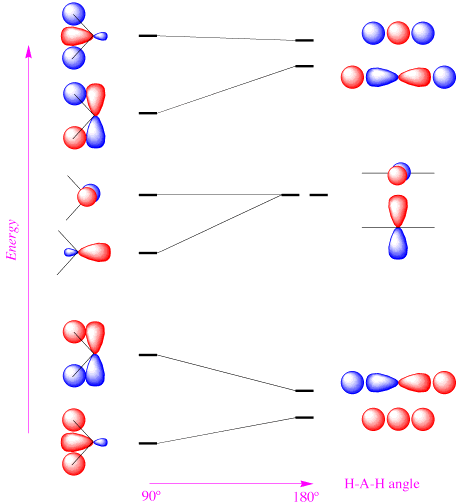# Homework 2

Name: ______________________________

Section: _____________________________

Student ID#:__________________________

## Q1

Normalize the following molecular orbital (on two different atoms)

$ψ = ψ_{s,A} +λψ_{s,B}$

where $$ψ_s$$ and $$ψ_{s,B}$$ are normalized and $$λ$$ is a parameter. Use the notation $$S$$ for overlap integral to simplify the result.

## Q2

The Cl−Sn−Cl bond angle in $$SnCl_2$$ is close to 90°. What does this imply about the hybridization of the the central tin atom?

## Q3

If the unnormalized hybrid orbitals in water that that describe bonding are expressed as

$|\psi_A \rangle = N \left( 0.61 | 2p_z \rangle + 0.79 | 2p_x \rangle - 0.5 | 2s \rangle \right)$

$|\psi_B \rangle = N \left( 0.61 | 2p_z \rangle - 0.79 | 2p_x \rangle - 0.5 | 2s \rangle \right)$

1. Use these expressions calculate the $$H-O-H$$ bond angle in the water molecule.
2. How does this compare with the experimental values?
3. How would the bond angle change if the bonding wavefunction were using non-hybridized atomic orbitals?
4. Calculate the s and p characters of the two wavefunctions.
5. Why isn't the $$| 2p_y \rangle$$ atomic orbital also mixed into the hybrid orbitals?

## Q4

What are the key aspects that separate valence bond theory from molecular orbital theory?

## Q5

Demonstrate that each of the three $$sp^2$$ orbitals satisfies the orthonormality criteria for wavefunctions.

## Q6

Determine the valence electron configurations and bond orders in the following homonuclear diatomics: $$C^+_2$$, $$C_2$$, $$C^−_2$$, $$N^+_2$$, $$N_2$$, $$N^−_2$$, $$O^+_2$$, $$O_2$$, $$O^−_2$$. Which species ones are paramagnetic?

## Q7

Consider the Walsh diagram for $$AH_2$$ molecules:1. How many valence electrons does the $$NH_2^+$$ ion have?
2. According to the Walsh diagram, would you expect $$NH_2^+$$ to be linear or bent?
3. How many valence electrons does the $$BH_2^+$$ ion have?
4. According to the Walsh diagram, would you expect $$BH_2^+$$ to be linear or bent?